This application note will use Simply Modbus software to communicate through Modbus RTU with the Roboteq controller. To connect the controller with PC through RS485 that Modbus RTU is using, a RS485 to USB converter will be required. Once the controller can communicate with Simply Modbus then it can be assumed that the controller can communicate with any other device supporting the Modbus RTU protocol.

1. Hardware connections

Connect the RS485 adapter according to the below diagram2. Roborun Configuration

• Enable the RS485 RTU mode
• Configure the ID of the slave device
• Leave the other configurations as they are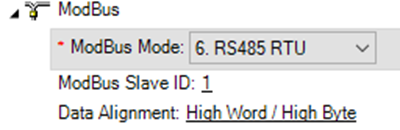3. Modbus RTU frame

The Modbus RTU frame has the following structure:Node ID: The ID of the slave. This is configured on the controller side

Function code: To read input Registers use 4. To write multiple holding registers use 10. The values are in HEX

Register address: Can be found from the commands dictionary. Note that the channel parameter is added to the command. So, 2001 register will give the motor amps of channel 1 while 2002 register will give the motor amps of channel 2

Number of registers: This depends on the length of the shift register. In Roboteq the registers used are always 32 bits

CRC: This is calculated and added by the Modbus software to the RTU frame

4. Simply Modbus Examples

On RoboteQ side give VAR 1 the value of 1234On Simply Modbus configure the below settings:Values explanation:

01:   Node ID. Configure according to the controller settings

20C1: The command ID + the index. Here it is 20C0+1, since we want to read VAR 1

0002: Number of registers to read. In Roboteq implementation all registers are 32 bits, so 2 registers of 16 bits should be read.

2BF7: CRC code calculated by Simply Modbus

Response:We can see that the value in the results field is 1234 in decimal.

On Roboteq, query the battery voltage to have it as a reference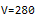Configure Simply Modbus as below: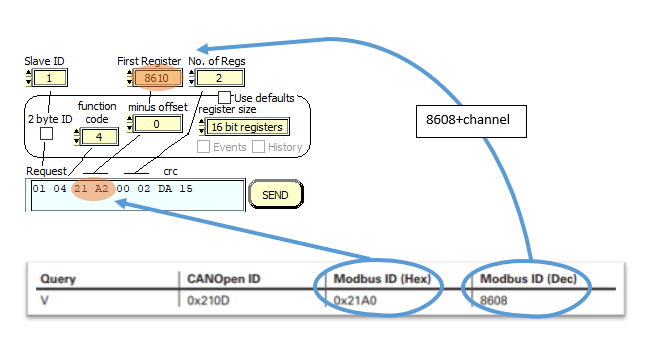Values explanation:

01:   Node ID

21A2: The command ID + the channel. Here it is 21A2+2, since we give an index of 2 in V parameter to get battery voltage

(?V 2)

0002: Number of registers to read. In Roboteq implementation all registers are 32 bits, so 2 registers of 16 bits should be read.

DA15: CRC code calculated by Simply Modbus

Response: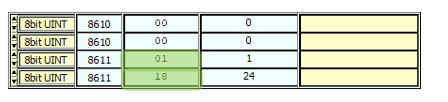The response is 0118 in hex, that is 280 (28V) in decimal.

4.2 Write multiple Registers

At Simply Modbus, to use write function, you must open the separate write tab.

Write Var 1

To write the value of 1235 on Var 1, the write window should be configured as follows:Values explanation:

01:   Node ID

10: Write function code (value in Hex)

01A1: The command ID + the channel. Here it is 01A0+1, since we want to write VAR 1

0002: Number of registers to read. In Roboteq implementation all registers are 32 bits, so 2 registers of 16 bits should be read.

04: Number of bytes to write

0000: Most significant bytes of the data to write

04D3: Less significant bytes of the data to write. Value is 1235 in decimal

7B46: CRC code calculated by Simply Modbus

When we query the VAR 1 from the controller, we confirm that its value has changed: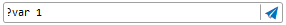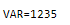Give motor command

To give the G command you need to configure the following: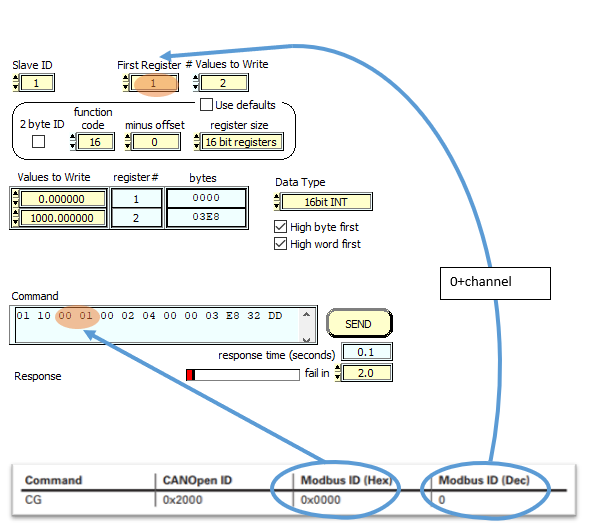Values explanation:

01:   Node ID

10: Write function code (value in Hex)

0001: The command ID + the channel. Here it is 0+1, since we want to write G command

0002: Number of registers to read. In Roboteq implementation all registers are 32 bits, so 2 registers of 16 bits should be read.

04: Number of bytes to write

0000: Most significant bytes of the data to write

03E8: Less significant bytes of the data to write. Vale is 1000 in decimal

32DD: CRC code calculated by Simply Modbus